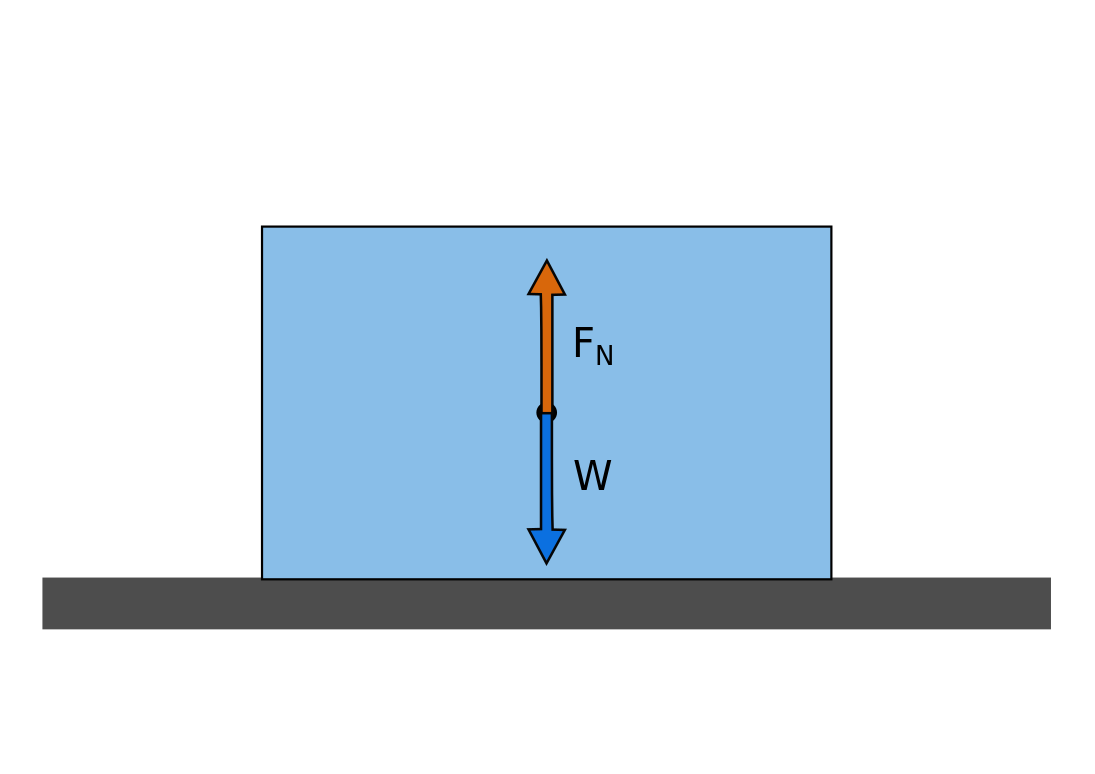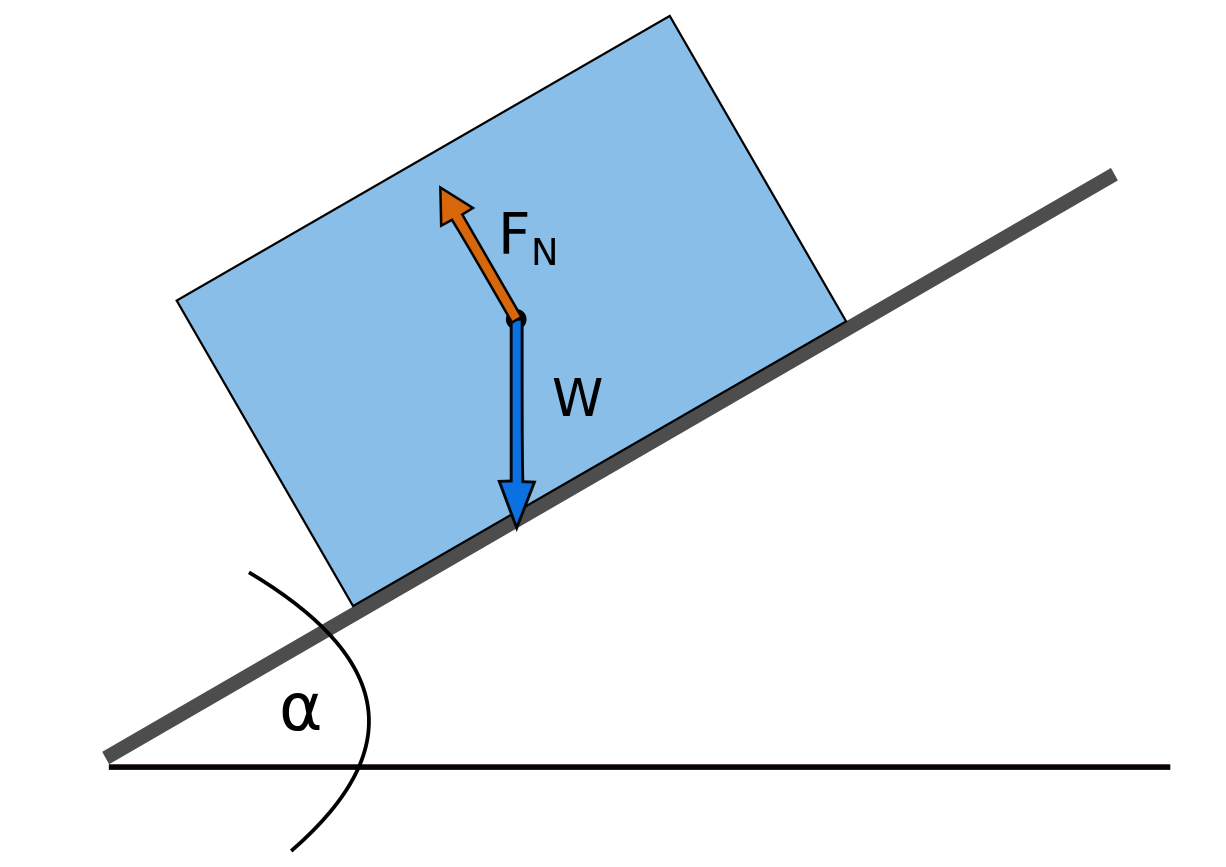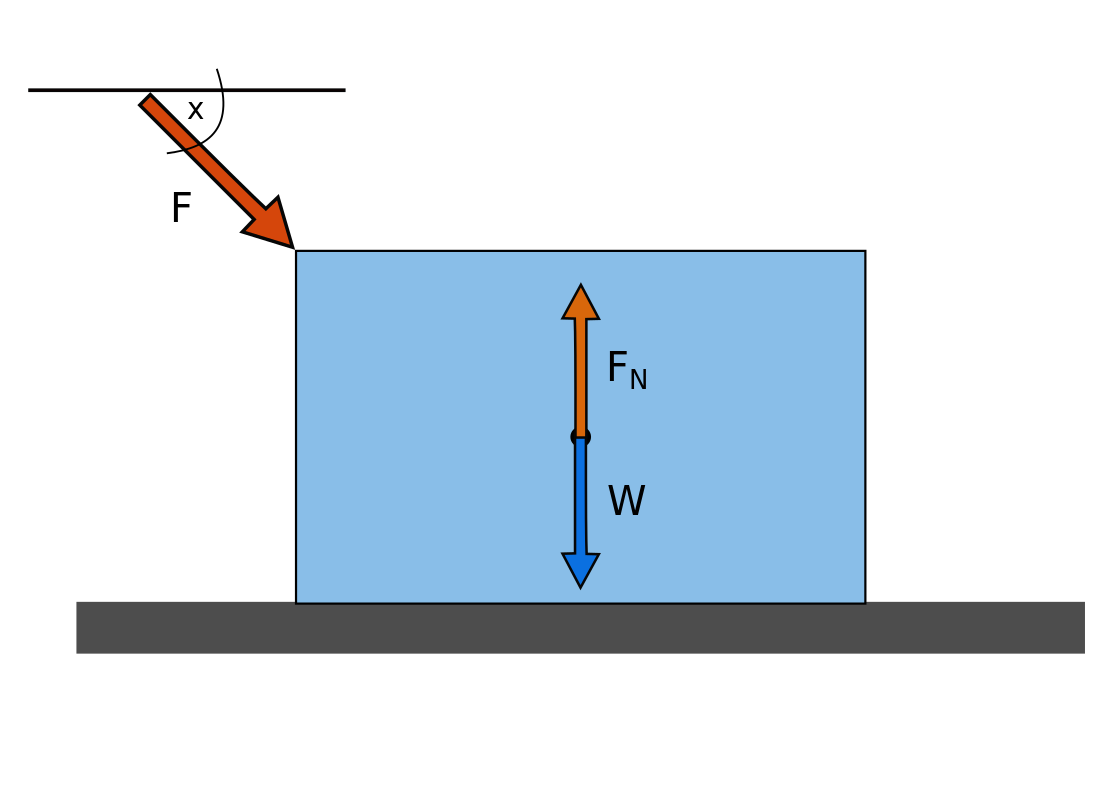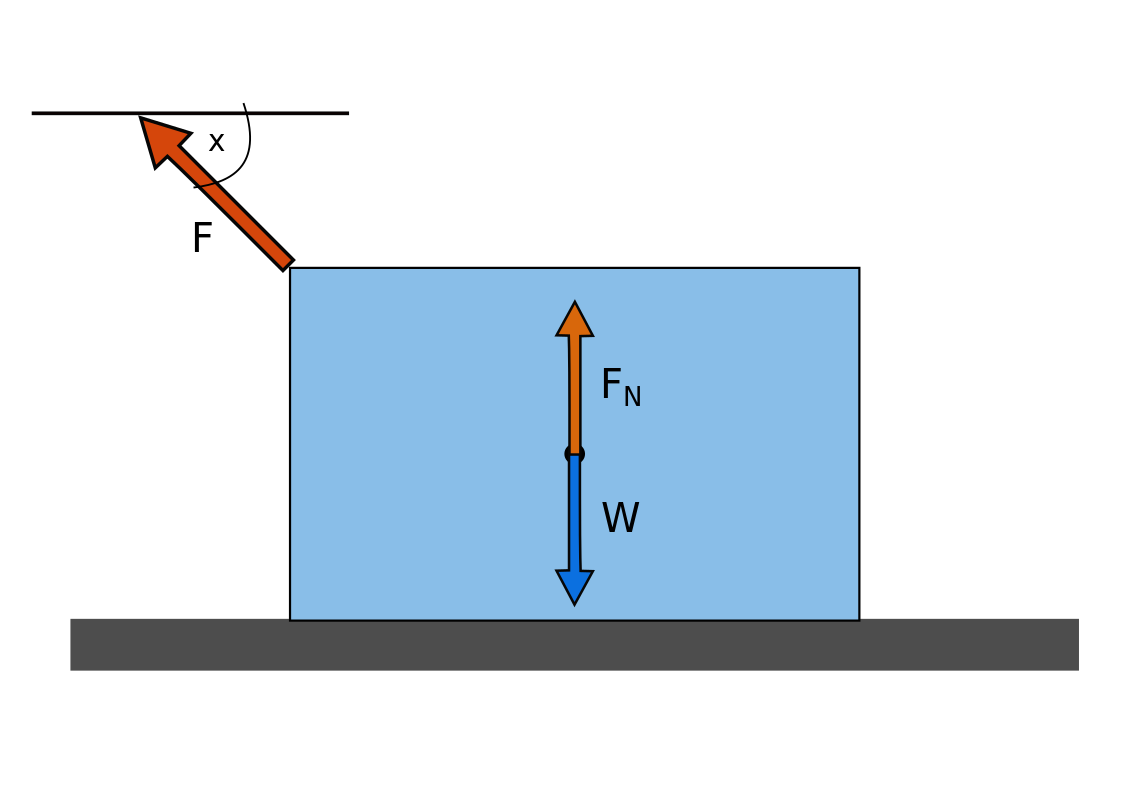# Normal Force Calculator

Created by Julia Żuławińska
Reviewed by Bogna Szyk and Jack Bowater
Last updated: Feb 02, 2023

The normal force calculator helps you find the force that a surface exerts to prevent an object from falling through it. In the following text, we will provide you with some normal force formulas, and an answer to the simple question: what is normal force? In doing so, we will also mention Newton's third law of motion. Scroll down to find out how to calculate normal force.

Since you're here, you might also enjoy our Newton's second law calculator.

## What is normal force?

Normal force is the perpendicular force that the surface exerts on an object. For example, if you put a book on a table, there is a gravitational force that is pulling it toward the ground. To counteract this force, the table exerts a force on the book, preventing it from falling. This counteracting force is called the normal force, and is represented by $\footnotesize F_N$, or $\footnotesize N$. The unit for the normal force is 'N' (Newton).

The normal force is a typical example of the Newton's third law of motion.

If one object exerts a force on a second object, the second object exerts a force of equal magnitude and opposite direction on the first object (action equals reaction).

So, a normal force is equal to the force exerted by the object on the surface. It's formulas vary with the slope of the surface.

1. For an object lying on a flat surface, the formula is:

$\footnotesize F_N = m ⋅ g$

where

• $\footnotesize m$ is the mass of an object.
• $\footnotesize g$ is the gravitational acceleration.

According to Newton's third law, the normal force ($\footnotesize F_N$) for an object on a flat surfaces is equal to its gravitational force ($\footnotesize W$).1. For an object placed on an inclined surface, the normal force equation is:

$\footnotesize F_N = m ⋅ g ⋅ cos(\alpha)$

where

• $\footnotesize \alpha$ is the surface inclination angle.On an inclined surface (assuming that the object doesn't slide down), the weight of the object is supported by both the normal force and friction. The gravitational force of the object is not opposite and equal to the normal force, but one of the force of gravity's vector's components is.

To find out how to calculate normal force with friction, use the friction calculator.

For objects on a flat surface, the normal force is counter to the objects weight. (Don't confuse it with mass! Weight is the same as gravitational force.) That is only the case when there is no outside force acting on the object, or, if there is, the outside force is parallel to the surface. Let's see what happens if there is an external force that isn't concurrent to the surface!

## Normal force formula with an external force

In calculations including an external force, you should only take into consideration the parallel vector component. That's why, in the normal force equations listed below, angles are included.

1. External Downward force$\footnotesize F_N = m ⋅ g + F ⋅ sin(x)$

,where:

• $\footnotesize F$ is the value of the external force.
• $\footnotesize x$ is the angle between the surface and the outside force.
1. External Upward Force$\footnotesize F_N = m ⋅ g - F ⋅ sin(x)$

If there is an outside force that is directed downwards, you need to add its vector component to the weight of the object. This increases the normal force, the outside force is pushing the object into the ground. It's the opposite case for an external force directed upward. It pulls an object away from the ground, so the normal force decreases.

If the force is directed straight upwards and equal to the gravitational force, the normal force is zero. Why? Because it counteracts the force of gravity entirely.

## How to use normal force equation - an example

How to find normal force on your own? Imagine there is a box lying on the ground that you want to move. It weights 100 kg. You push on it at a 45 degrees angle with 250 N of force.

$\footnotesize F_N = 100 ⋅ 9.807 + 250 ⋅ sin(45\degree) = 980.7 + 250 \frac{\sqrt2}{2} = 1157.4 N$

The ground exerts 1,157.4 N of force on the box. If the box was on a soft surface, because of your additional force, it might collapse. That's way, to save strength, it's best to push things by their side, straight toward the destination.

Have you enjoyed our normal force calculator? Check out the work and power calculator too!

## FAQ

### How to find normal force on an incline?

To find the normal force of an object on an incline, you need to:

1. Find the mass of the object. (It should be in kg.)
2. Find the angle of incline of the surface.
3. Multiply mass, gravitational acceleration, and the cosine of the inclination angle.
Normal force = m x g x cos(α)
4. You can check your result in our normal force calculator.

### What's the normal force exerted on a 0.6 kg book?

It's 5.884 N. To find the normal force of a book lying on a horizontal surface, you need to multiply the book's mass (0.6 kg) and gravitational acceleration (9.807 m/s² ).

### Are normal force and weight always equal?

Normal force and weight aren't always equal. They're equal when:

• There are no other forces exerted in the vertical direction; and
• An object is on a flat surface.

Normal force and weight are not equal when:

• There's an additional force that at least in part works in the vertical direction; or
• The object is on an inclined surface.

### Can normal force be negative?

Yes, the normal force can be negative. Forces are vectors, so it's up to us to decide in which direction they will be positive and negative. If an object is lying on a flat surface, and we assume that weight is positive, then normal force will be negative.

Julia Żuławińska
Surface
InclinedMass
kg
Angle
deg
Normal force
N
People also viewed…

### Bridge rectifier

You can use this bridge rectifier calculator to compute various rectification attributes of the conversion of AC (alternating current) into DC (direct current).

### Meat footprint

Check out the impact meat has on the environment and your health.